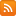CONTÁCTENOS - 91 575 78 24Estás en www.ingenieriayarte.com
Si no encuentra un libro lo buscamos por Ud.
91 575 78 24

## CESTA DE LA COMPRA

Tiene 0 productos en su cesta Importe total: 0
Modeling and Computing for Geotechnical Engineering: An Introduction

# Modeling and Computing for Geotechnical Engineering: An Introduction

## Descripción

Modeling and computing is becoming an essential part of the analysis and design of an engineered system. This is also true "geotechnical systems", such as soil-foundations, earth dams and other soil structure systems

## Características

• ISBN: 9781498771672
• Páginas: 492
• Tamaño: 17x24
• Edición:
• Idioma: Ingles
• Año: 2018

Compra bajo pedidoDisponibilidad: 3 a 7 Días

# Contenido Modeling and Computing for Geotechnical Engineering: An Introduction

•The book includes MATLAB codes and MAPLE worksheets of simple analytical and numerical solutions of important daily life geotechnical problems with powerful and aesthetic visualization features in multi-D situations
•Teaches the fundamentals of analytical and numerical methods used to develop those solutions
•Serves as a basic textbook to a wide range of reader profiles from graduate students to researchers and engineers by combining the necessary theory and related practical applications

Summary

Modeling and computing is becoming an essential part of the analysis and design of an engineered system. This is also true "geotechnical systems", such as soil-foundations, earth dams and other soil structure systems. The general goal of ‘modeling and computing’ is to predict and understand the behaviour of the system subjected to a variety of possible conditions/scenarios (with respect to both external stimuli and system parameters), which provides the basis for a rational design of the system. The essence of this is to predict the response of the system to a set of external forces. The modelling and computing essentially involve the following three phases: (a) Idealization of the actual physical problem, (b) Formulation of a mathematical model represented by a set of equations governing the response of the system, and (c) Solution of the governing equations (often requiring numerical methods) and graphical representation of the numerical results. This book will introduce these phases.

MATLAB® codes and MAPLE® worksheets are available for those who have bought the book. Please contact the author at mbulker@itu.edu.tr or canulker@gmail.com. Kindly provide the invoice number and date of purchase.

Preface

Introduction

BASIC MECHANICS

Stresses and Strains

Introduction

Reference Coordinate System: Notations

Strains

Stresses

Mohr’s Circle

Physical Laws and Governing Equations

Introduction

Idealizations

Total and Effective Stresses in Soils

Law of Conservation of Momentum: Equilibrium Equations

Law of Conservation of Mass

ELEMENTAL RESPONSE: CONSTITUTIVE MODELS

I. Introduction

II. Soil Behavior: From Experimental Results

III. Modeling of Soil Behavior

Elasticity

Elastic Constitutive Law

Plasticity Theory: Nonlinear Deformation of Soils

Introduction

Nonlinear Deformation of Soils

Elements of Plasticity

Yielding Criteria

Post-Yield Behavior

Perfect Plasticity

Hardening Plasticity

Exercise Problems

Viscoelasticity and Viscoplasticity

Introduction

Viscoelastic Behavior: Fundamental Rheological Models

Viscoelastic Behavior: Composite Rheological Models

Formulation Methods in Viscoelasticity

Viscoplasticity

Exercise Problems

SYSTEM RESPONSE: METHODS OF ANALYSES

Analytical Methods

Introduction

1-D Flow through a Land Mass: Island Recharge Problem

Regional Groundwater Flow: Steady State Seepage

1-D Deformation of a Soil Column

1-D Consolidation of a Soil Column: Decoupled Flow and Deformation

Contaminant Transport

1-D Coupled Flow and Deformation

2-D Coupled Flow and Deformation

Exercise Problems

Semi-Analytical Methods

Introduction

Stress Analysis

Quasi-Static Analysis of Multi-Layer Porous Media under Waves

Exercise Problems

Finite Difference Method

Introduction

Finite Difference Approximation of Derivatives

FDM for Consolidation (Parabolic) Equation

FDM for Seepage (Laplace) Equation: 2-D Steady State Flow

FDM for Groundwater Flow: Aquifer Simulation

FDM for Consolidation of a Layered System

FDM for Laterally Loaded Piles: Soil-Structure Interaction

Error, Convergence and Stability

Exercise Problems

Finite Element Method

Introduction

Direct Stiffness Method

Galerkin Method of Weighted Residual

FEM: 1-D Problems

FEM: 2-D Problems

Basic Element Formulations

The Principle of Minimum Potential Energy

Isoparametric Element Formulation

Exercise Problems

Appendices

A.1 Fourier Series and Fourier Transform

A.2 Laplace Transform

A.3 MATLAB Commands: FFT, IFFT, FFTSHIFT

A.4 Solution Flow Chart for the Analysis of a Viscoelastic Material

A.5 Analytical Solution of Wave-Induced Porous Soil Layer Response

A.5 Semi-Analytical Solution of Wave-Induced Multi-Layer Porous Soil Response

References

Index

## ACERCA DE LA EMPRESA